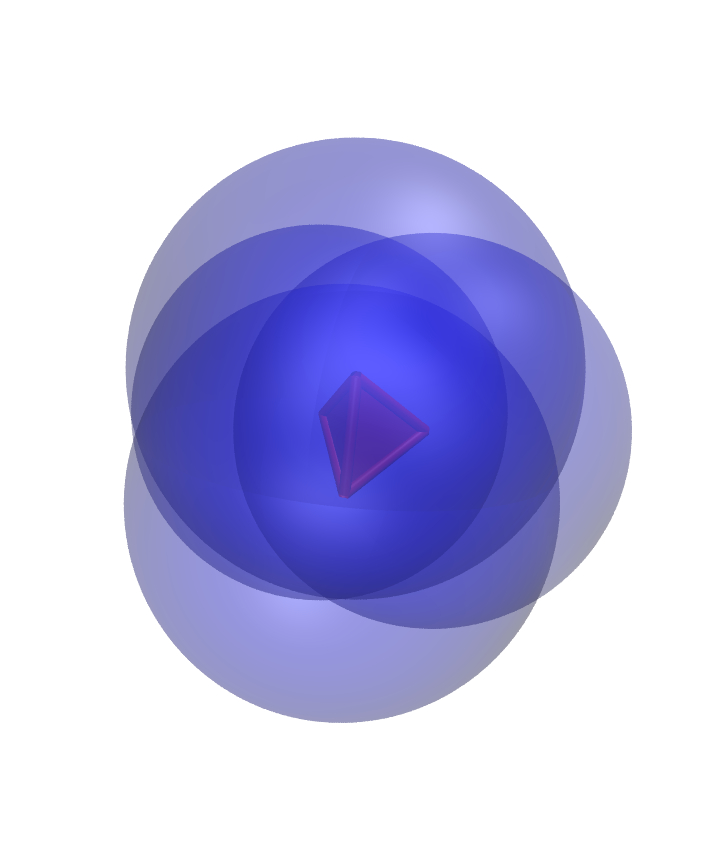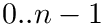Structural Bioinformatics Library Template C++ / Python API for developping structural bioinformatics applications.
User Manual# Alpha_complexes_extensions

Authors: F. Cazals and T. Dreyfus

# Introduction

The 3D weighted-complex is intensively used in the SBL library for modeling molecules. This package provides two major tools for:

• visualizing a 3D weighted-complex using molecular viewers,
• serializing a 3D weighted-complex.

These functionality are provided for the 3D weighted-complex data structures of the CGAL library, namely CGAL::Alpha_shape_3 and CGAL::Fixed_alpha_shape_3.

# Functionality

## Visualization

It uses the package Molecular_viewers for creating input files of molecular viewers such as PyMOL or VMD for visualizing the simplices of a 3D weighted-complex, except the tetrahedra. The package Molecular_viewers works as follows :

• (i) open a file,
• (iii) output the 3D weighted-complex using the output stream operator on the viewer.

There are two ways to output a 3D weighted-complex:

• by iterating over each simplex, and dumping each simplex; it will output all simplices of the underlying triangulation whatever is the status of the simplex in the-complex.
• by dumping directly the-complex; it will output only the edges and faces of the underlying triangulation that are in the-complex.

See Visualization with VMD for an example.

## Serialization

It uses the Boost serialization library for serializing the vertices of the-complex. More precisely, each vertex of the-complex can be serialized exactly as the vertex of the underlying triangulation, as defined in the package Triangulations_extensions_3.

See Serializing the vertices for an example.

# Examples

## Visualization with VMD

The following example load a plain text file of 3D spheres, compute the-complex of the input, and save in a visualization state file for VMD the vertices, edges and faces of the-complex. Note that the vertices are represented as 3D transparent red spheres, while the other simplices are represented with opaque red geometric objects.

## Serializing the vertices

The following example load a plain text file of 3D spheres, compute the-complex of the input, and save in an archive the vertices of the output-complex.